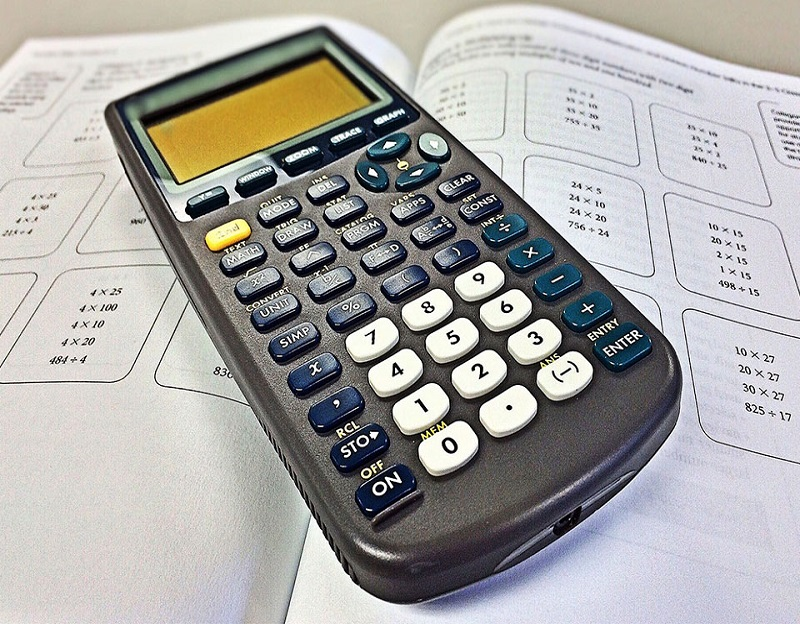# Can Calculators Affect Students’ Calculating Capacity Adversely?

Mar 13, 2020This is a pretty common question raised by many math teachers, tutors and parents. Are calculators really necessary for students? This is a pretty difficult question to answer because you might not agree with us. So we have decided to highlight a few pros and cons of using calculators while doing math from a very early age. After that, we’ll provide you with an answer to the question above. You can then decide whether we are correct or not. Let’s begin.

## Pros

### Technical knowledge

In this ever-advancing technology oriented world, technology has also becomes a part of a student’s classroom ranging from iPads to laptops. A calculator is also a very part of this technology. Therefore, it’s really good for a student to understand how calculator works.

A calculator is usually a pretty simple instrument and it is necessary that a student is able to use it effectively throughout his/her career for various operations. Calculators can be used by a young student to set his/her monthly expenditure according to the pocket money, do various operations and other math-related tasks. Therefore, it is definitely beneficial for them to start early on calculators purely on the basis of this advantage.

### Enjoyment

Children do love technology. The world of computers, smart phones, apps, games look very enticing from a student’s point of view. A scientific calculator does have a somewhat-similar effect, at least up to a certain level. A student feels overjoyed to use a calculator while doing their math homework.

A simpler way to explain this is that- students love to press buttons. And calculators do have buttons. Therefore, calculators are fun.

### Accuracy

This point is pretty obvious, to be honest. Using calculators can highly improve the math accuracy. The main reason behind a poor math performance is silly mistakes centered on basic arithmetic operations. A calculator can solve that problem to a great extent. Additionally, calculators are fast enough to give you answers within the blink of an eye.

## Cons

### Complacency

Students will be able to perform various math operations quickly and effectively with calculators like we said before. That’s definitely an advantage but at the same time, it can also be a disadvantage when certain students become complacent enough with their math homework because they are actually solving their sums way before schedule.

Students must know about the ways through which they can do basic arithmetic operations by hand. Teachers and parent must remember that to check the overuse of calculators in assignments.

### Cheating

Graphing calculators are advanced calculators used to perform various advanced calculations other than basic arithmetic operations namely additions, subtractions, multiplications, divisions. That’s fine from our end. The problem is these calculators also provide the users with the advantage of storing notes in their memory. Thus, you can’t deny the chance of misusing this advanced feature of the graphing calculator.

Any student can store some math-related information in his/her respective calculator in a math test that allows the use of calculators during the exam. Such information may range from formulae to rules.

It becomes too difficult for the teacher actually to notice this during exams because they are already permitted to sue calculators in classes. Therefore, it’s recommended that teachers introduce a calculator policy in classes; meaning students cannot use calculators all the time, only at specific times according to the teacher and not during exams unless they are at a higher secondary level. It’s also advisable to implement regular calculators in classes rather than the advanced ones for this problem.

### Cost

One should always consider the cost factor when a thing in question is a piece of technology. Most will say that calculators are dirt-cheap and hence, feasible enough. Well, it’s not entirely true. Advanced graphing calculators can burn a hole in your pocket. And then the question of batteries does creep in as well. To sum up, it’s an investment and hence, a con.

## Our verdict

We reckon students should NOT use a calculator to perform their basic operations WHEN they are at an early stage of their career. Let them take their time to come to a result because that’s how they’ll learn; that’s how they can gain the math fluency.

Another thing to remember is that many competitive exams do not allow the use of calculators. It’s, therefore, necessary for students to practice sums by hand otherwise; they will be under immense pressure in the examination hall. Students can refer to various short-cut tricks and techniques from professional math tutors to help them out in such exams where time is of the essence. We’ll conclude this article for now. Hope you had a good read.